#### Recommend to LibarianSubmit your articleView all articles

Volume 1 Pages 1-71

Volume 2 Pages 1-66

Search this journal

###### How to Cite?
ES Materials & Manufacturing

DOI:10.30919/esmm5f756

ES Materials & Manufacturing, 2020, 9, 34-39

Published online: 04 Jun 2020

Received 18 Apr 2020, Accepted 04 Jun 2020

# Machine Learning Prediction for Bandgaps of Inorganic Materials

Lang Wu2, Yue Xiao1, Mithun Ghosh2, Qiang Zhou2, Qing Hao1,*

# 1 Department of Aerospace and Mechanical Engineering, University of Arizona, Tucson, AZ 85721 U.S.A

2 Department of Systems and Industrial Engineering, University of Arizona, Tucson, AZ 85721 U.S.A

*Corresponding authors: Qing Hao ([email protected])

Table of ContentA new machine learning method is used to predict the bandgaps of inorganic compounds using known compositional features.

## Abstract

Machine learning approaches are explored to predict the bandgaps of inorganic compounds using known compositional features, based on a dataset of 3,896 compounds with experimentally measured bandgaps. Particularly among various existing methods, we propose a new method, random forest with Gaussian process model as leaf nodes (RF-GP), and show its advantages. We have also investigated ensemble learning methods, which produce superior results to other traditional machine learning methods, but at the cost of extra computational load and further reduced interpretability.

## 1. Introduction

In recent years, machine learning-based predictions for material properties have gained growing interests. Compared with the time-consuming experimental inferences and computational methods like density functional theory (DFT) or molecular dynamics (MD), machine learning-based predictive algorithm provides a high-throughput, computationally inexpensive way to render material properties. Specifically, a model to predict electronic properties such as the bandgap can provide an important guidance in the search of the ideal materials, which will benefit fields such as solar cells, and heterojunction optical devices, etc.  In these applications, a precise bandgap is necessary for the evaluation of the device performance. Through machine learning, the correlation between certain material properties and attributes may be found and eventually leads to the discovery of new materials.[5,6]

Many recent studies have used machine learning algorithms to help with analysis in material science areas. For example, Wang et al. introduced a self-adaptive differential evolution algorithm to optimize a reduced mechanism of 2-Butanone, whose performance is similar to those of the detailed mechanism. Peng et al. used the random forest algorithm to analyze the importance of various factors on solar evaporation. Zhang et al. provided an overview of machine learning methods for screening of the thermal conductivity for compounds, composites and alloys.[ Wan et al. reviewed the research progress regarding the materials discovery and properties prediction in thermal transport via materials informatics.

For bandgap predictions, Pilania et al. adopted a linear least square regression combined with kernel ridge regression (KRR) to predict bandgaps of double perovskites. The KRR can incorporate complex non-linear relationships between different material features, but this model can only be applied to a limited chemical space for nonmagnetic perovskites. Ward et al. adopted a random forest technique with a categorized dataset based on properties such as the range of bandgaps and element groups. However, the partitioning of the materials has lowered the robustness of the model, though the prediction accuracy is relatively high.

In this work, we investigate machine learning techniques to recognize meaningful patterns in bandgap values across thousands of compounds and their chemical properties. To represent the materials in a feature space, the chemical composition-based approach is adopted. As an advancement to previous studies, we propose a new method by combining the random forest and Gaussian process (RF-GP), which exhibits some advantages over existing methods.

## 2. Data Description

Our dataset employs 3896 experimentally reported bandgaps of inorganic compounds composed of 2458 unique element compositions, which were extracted from the literature[13-17] as referenced. The histogram of bandgaps of these materials is shown in Fig. 1.  It illustrates that the compositions used for training the models range from small bandgap semiconductors such as CrSb2 (Eg = 0.16 eV) to ultra-wide bandgap materials like LiF (Eg =11.7 eV). Inputs to models termed as features (in machine learning) or descriptors (in materials science) should be easily accessible properties, obtained by experiments and simple computations. In the rest of this paper, model inputs will be called “features.” The applied machine-learning models predict the bandgap using a feature set based only on the elemental properties of the constituent elements, which are related to the atom’s relative position on the periodic table, the electronic structure, and their physical properties. The full list of variables is provided in Table A1 (refer to supporting materials). The feature set is limited to composition features in machine-learning models because there is currently no simple and effective way to describe crystal structures. Also, we find out the detailed atomic structure may not be needed to achieve certain accuracy for the prediction.Fig. 1 Bandgap distribution of 3896 inorganic compounds in our dataset.

## 3. MethodsFig. 2 Overall workflow.

The methods utilized in this study are linear Lasso (Least Absolute Shrinkage and Selection Operator) and Ridge regression, random forest, support vector regression (SVR), Gaussian process regression (GPR) and artificial neural network (ANN). These techniques are briefly summarized here, with the connection between the techniques shown in Fig. 2.

3.1 Ridge and Lasso regression

Linear regression is the most common statistical method for predictive modeling. Ridge and Lasso are two advanced linear regressions with regularization terms, which are used to prevent over-fitting and reduce model complexity. As is shown in Equation (1), in ridge regression, the cost function is modified by applying an L2 norm as a penalty such that the cost function is penalized if the coefficients are large. That means ridge regression shrinks the coefficients and helps to overcome multicollinearity. Similarly, Lasso regression adds the magnitude of the coefficients (L1 norm) as a penalty instead of taking the square of the coefficients. This type of regularization can cause some coefficients to be exactly zero, namely, some of the features are completely neglected for the evaluation of output. Therefore, Lasso regression helps not only in reducing over-fitting but also in feature selection. In Equations (1) and (2), X represents the matrix of input features, y is the actual bandgap values and the terms λ1,λ2 are tuning parameters.(1)(2)

3.2 Artificial neural networksFig. 3 Artificial neural network illustration.

One of the principal tools used in machine learning is artificial neural networks (ANN). As the “neural” part of the name implies, they are computing systems inspired by mimicking the way the biological nervous system processes information. ANN uses multiple layers of nodes, each layer being completely connected to the next, as shown in Fig. 3. The input layer is composed of a group of neurons representing the input features. Each neuron in hidden layers is a result of a weighted sum of the neurons in the previous layer followed by an activation function whose motive is to introduce non-linearity into the model. Depending upon the type of problem (classification or regression), the output layer can have one or multiple nodes that collect the processed information from the last hidden layer. ANN becomes popular in the last several decades due to the arrival of back propagation, which allows the network to adjust the connection weights after each batch of data is processed, based on the difference between the actual value and the predicted value.

3.3 Support vector regression

Different from the support vector machine (SVM, a classification algorithm), support vector regression (SVR) is used to predict a continuous variable. Unlike other linear regression models that aim to reduce the difference between the true and the predicted value, SVR attempts to match the best hyperplane (which is a line used to predict the continuous value in Fig. 4) within a predefined threshold value denoted as ε. As is shown in Fig. 4, without violating the margin, the tube attempts to fit as many data points as possible. The error threshold controls the width of the boundary. Specifically, the objective function and constraints can be formulated as Equation (3):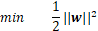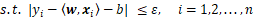,      (3)

where <. , . >denotes the inner product, ε is a tuning parameter served as an error threshold, and xiis a training sample with a target value yi. The inner product plus intercept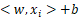is the prediction for that sample. The constraints in Equation (3) means that the errors between all predictions and target values need to be within the ε range. ||w|| is minimized to make the fit as flat as possible. Slack variables may be added into the above model in case that there is no feasible solution for the above optimization problem. Furthermore, when the dataset is not linearly separated, a kernel function might be applied to transform the data into a higher dimensional feature space where linear separation is possible, which is called non-linear kernel SVR. The radial basis function kernel will be used in the subsequent SVR model.

3.4 Random forest

Decision trees learn hierarchically by continuously dividing training samples into branches that maximize the information gain of each split. This branching structure allows decision trees to naturally learn non-linear relationships. Random Forest is an ensemble method that fits classifying decision trees on subsets of a dataset and averages over the trees to improve prediction accuracy and reduce over-fitting. During model training, the samples of each tree are drawn with replacement, known as bootstrapping, which means that some samples may be used multiple times in a single tree. Sampling with replacement decreases the variance of the model but not at the cost of increasing bias. When splitting a node in the tree, a random subset of the features is used to create the best split.

3.5 Gaussian process regression

Gaussian process (GP) regression model[22,23] has been increasingly popular for the prediction of various materials properties, with the capability of handling complex non-linear relationships. The GP regression conducts a measure of the distance similarity (the kernel function) between samples to predict the value for an unobserved instance. The prediction is not just an estimate for that sample but also includes uncertainty information. In the present case, the commonly used radial basis function kernel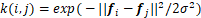is adopted to measure the similarity of materials properties. It is significant to construct features where materials have a small “distance” if their properties are similar.

3.6 Random forest with GP node

GP is attractive to be used due to its accommodation of the prior knowledge and estimates of predictive confidence. However, the bandgap training data tend to be nonstationary (i.e., statistical properties of data are not constant in the feature space), which is difficult for standard GP to handle. Partitioning the data into smaller subregions (that are relatively stationary) is a simple and effective way to deal with data non-stationarity. This can be easily achieved by a tree-based algorithm such as the random forest. Hence, we combine GP with random forest and propose an RF-GP method (random forest with Gaussian process models as leaf nodes). Unlike standard random forest that uses simple average values for leaf nodes, the RF-GP uses GP, which is a much more powerful nonlinear regressor. Typically, a well-performed random forest has only a small number of data points at each leaf node (e.g., two or three), but the RF-GP can handle much larger leaf nodes due to the nonlinear regressor GP. As larger leaf nodes correspond to larger subregions in the feature space, this potentially increases the interpretability of the model as well. Specifically, the tree partitioning approach will do binary splits at each node based on a randomly selected feature and its critical value (e.g.,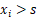). For each way of splitting the data into two subsets based on the selected feature, two GP models can be fitted (one for each subset). The critical value (and hence the optimal split) is determined by minimizing the sum of prediction errors of the two GP models.

## 4. Results and Discussion

In this section, we discuss the results of all methods described in the previous section. The dataset is randomly split into a training and testing set with a ratio of 9:1. For each method, we perform 10-fold cross-validation on the training set to optimize the hyper-parameters of the model and then report the root mean squared error (RMSE), mean absolute error (MAE) and coefficient of determination (R2) on the testing set. These three metrics are described as follows: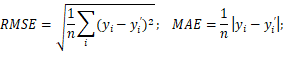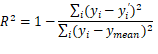where yi, y­i',ymean and n represent the true bandgap, predicted bandgap, mean of all true bandgaps and the number of training samples, respectively. The R2 score ranges from 0 to 1, with 1 being the perfect performance.Fig. 4 Linear SVR illustration.

Table 1. MAE, MSE and R2 scores for different machine learning methods.

 Model MAE RMSE R2 Linear Lasso 0.569 0.750 0.708 Linear Ridge 0.481 0.642 0.786 Random forest 0.255 0.419 0.909 SVR 0.395 0.566 0.833 GPR 0.314 0.552 0.841 ANN 0.529 0.723 0.728

Table 1 presents the MAE, RMSE and R2 scores of bandgap predictions with different machine learning methods using the same features set. Here, the minimum number of samples required to be at a leaf node of the random forest is two. From Table 1, it can be seen that the random forest method has the best prediction performance compared with other machine learning methods. One reason may be that it has its built-in feature selection architecture. The random forest consists of several decision trees. Every node in the decision trees is a condition on a single feature, designed to split the (sub)dataset into two so that similar response values end up in the same set. Therefore, the importance of each feature is derived from how “pure” each of the sets is, by which random forest selects the important features. The Lasso method also includes feature selection, but it does not work very well likely because the bandgap and features are not much linearly related.

Fig. 5 illustrates the bandgap predictions versus experimental results on the testing set. It shows that the machine learning-based predictions are, in general, close to experimentally measured bandgaps. However, it is not hard to observe that the solid dots with wide bandgaps (>5.0 eV) often fall below the dashed line, which means that there is often underestimation for the wide bandgap compositions. It is likely due to a limited number of compounds in the dataset with these very wide bandgaps.

4.1 Comparisons of RF and RF-GP performance

From Table 1 and Fig. 5, it is observed that the random forest method has the best prediction performance. Now we compare the random forest with the proposed RF-GP method, which may be viewed as a more advanced version of random forest that has nonlinear regressors at leaf nodes. We compare the performance between the regular random forest (RF) and RF-GP with various minimal leaf node sizes.Fig. 5 Bandgap predictions on the testing set with different models. The red dashed lines represent the ideal results.

Table 2. RMSE comparisons between RF and RF-GP.

 Leaf node size RMSE for RF RMSE for RF-GP 10 0.510 0.520 20 0.548 0.544 30 0.587 0.511 50 0.642 0.530 60 0.665 0.535 80 0.733 0.550 100 0.799 0.555

Table. 2 shows that the performance of RF-GP is reasonably good even with large leaf nodes due to its nonlinear model. Unlike the RF, the performance of RF-GP does not increase monotonically as the node size decreases. This is because a GP needs to be fitted with a reasonable sample size due to its model complexity, which means there is an optimal node size for RF-GP. But overall, the performance of RF-GP is very robust to the node size and it is reasonably good even with a large node size. However, RF performance gets monotonically worse as the leaf node size increases due to its simple average predictor at the node (a better result in Table. 1 was achieved by a node size of 2). An added advantage of RF-GP is that it is more likely to identify better or even physically interpretable subcategories of the data due to its much larger leaf node size comparing with a regular RF.

4.2 Ensemble learning

In this section, we investigate more ensemble learning methods[24,25] on the material bandgaps dataset in light of the finding that ensemble-based random forest performs better in the previous study. Ensemble methods are proposed to decrease variance, bias or improve predictions by combining several machine learning techniques into one predictive model. In general, ensemble methods can be divided into three groups: bagging (bootstrap aggregation), boosting and stacking. Bagging uses bootstrap sampling to obtain data subsets for training base learners. For aggregating the outputs from base learners, bagging uses voting for classification and averaging for regression. The previous random forest (leaf node size = 2) as a bagging method is used here to make comparisons. Boosting has been proposed to transform weak learners (models that are only slightly better than random guesses, such as small decision trees) to strong learners. Samples that are misclassified by earlier rounds will be allocated more weights. The boosting algorithm applied here is XgBoost (Extreme gradient boosting). Stacking method is usually composed of two-layer algorithms. The inputs in the second-layer algorithm are the predictions generated by the first-layer machine learning algorithms. This second-layer algorithm is trained to optimally combine the model predictions to constitute a new set of outputs. For instance, the first-layer algorithms may consist of GP regression, random forest and SVR, whose predictions are combined by XgBoost as a second layer regressor.

The prediction performance by three ensemble methods is shown in Table 3. With ensemble learning and elemental features, it is interesting that all of the methods yield similarly good performance. This further emphasizes the entangled nature of composition and crystal structure. Besides, the stacking method shows slightly better performance as it integrates multiple machine learning methods.

Table 3. Comparisons of three ensemble methods on bandgap predictions.

 Models MAE RMSE R2 Random forest 0.255 0.419 0.909 XgBoost 0.249 0.408 0.912 Stacking ensemble 0.246 0.402 0.914

## 5. Conclusion

Ever since the advent of data science and efficient machine learning tools, they have been used by diverse fields to solve domain-specific problems. Together with the abundance of data, they provide a unique opportunity for many unsolved problems in materials science. In summary, this paper presents several supervised machine-learning schemes trained using 3896 experimentally measured bandgaps from the literature. Our results show that these methods, using a feature set based on only composition information, are capable of making bandgap predictions with reasonable accuracy. Among various existing methods, we propose a new method (i.e., RF-GP) that has its advantages. In addition, the ensemble learning models outperform other traditional machine learning methods, but at the cost of extra computa-tion and minimal interpretability. The results show that these machine learning methods can reliably predict bandgaps at a significantly reduced computational cost, compared with first-principles methods.

Two main directions we might further improve our model are: adding more training data and using feature engineering. Besides, adding material crystal structure into the feature set has the potential to further improve prediction accuracy. The proper representation of crystal structure information is, however, a challenging issue with many ongoing active types of research.

Acknowledgments

The authors thank the support from the Faculty Seed Grant at the University of Arizona. Q. H. also acknowledges the support from the National Science Foundation (grant number CBET-1651840) for materials studies.

Conflict of Interest

There is no conflict of interest.

Supporting Information

Applicable, https://dx.doi.org/10.30919/esmm5f756

References

 A. G. Kusne, D. Keller, A. Anderson, A. Zaban and I. Takeuchi, Nanotechnology, 2015, 26, 444002. doi: 10.1088/0957-4484/26/44/444002.

 A. Jain, S. Ong, G. Hautier, W. Chen, W. Richards, S. Dacek, S. Cholia, D. Gunter, D. Skinner, G. Ceder and K. Persson, APL Mater., 2013, 1(1), 011002. doi: 10.1063/1.4812323.

 J. Ma and L. Wang, Nature, 2016, 24924. doi: 10.1038/srep24924.

 P. Dey, J. Bible, S. Datta, S. Broderick, J. Jasinski, M. Sunkara, M. Menon and K. Rajan,Comp. Mater. Sci., 2014, 83, 185-195. doi: 10.1016/j.commatsci.2013.10.016.

 M. Gaultois, A. Oliynyk, T. Sparks, G. Mullholand and B. Meredig, APL Mater., 2016, 4, 053213. doi: 10.1063/1.4952607.

 B. Meredig, A. Agrawal, S. Kirklin, J. Saal, J. Doak, A. Thompson, K. Zhang, A. Choudhary and C. Wolverton, Phys. Rev. B, 2014, 89, 094104. doi: 10.1103/PhysRevB.89.094104.

 Y. Wang, S. Liu, J. Cheng, X. Xiao, W. Feng, N. Yang and C. Zuo, ES Mater. Manuf., 2019, 6, 28-37. doi: 10.30919/esmm5f615.

 G. Peng, S.W. Sharshir, Y. Wang, M. An, A.E. Kabeel, J. Zang, L. Zhang and N. Yang, arXiv preprint arXiv:1906.08461, 2019

 H. Zhang, K. Hippalgaonkar, T. Buonassisi, O.M. Løvvik, E. Sagvolden and D. Ding, arXiv preprint arXiv:1901.05801, 2019.

 X. Wan, W. Feng, Y. Wang, H. Wang, X. Zhang, C. Deng and N. Yang, Nano Lett., 2019, 19(6), 3387-3395. doi: 10.1021/acs.nanolett.8b05196.

G. Pilania, A. Mannodi, B. Uberuaga, R. Ramprasad, J. Gubernatis and T. Lookman, Sci. Rep., 2016, 6, 19375. doi: 10.1038/srep19375.

 L. Ward, A. Agrawal, A. Choudhary and C. Wolverton, NPJComput. Mater., 2016, 2, 16028. doi: 10.1038/npjcompumats.2016.28.

Y. Zhuo, A. Mansouri Tehrani and J. Brgoch,J.Phys. Chem. Lett., 2018, 9(7), 1668-1673. doi: 10.1021/acs.jpclett.8b00124.

N. N. Kiselyova, V. A. Dudarev and M. A. Korzhuyev, Inorg. Mater. Appl. Res., 2016, 7, 34-39. doi: 10.1134/S2075113316010093.

W. H. Strehlow and E. L. Cook, J. Phys. Chem. Ref. Data, 1973, 2, 163-200. doi: 10.1063/1.3253115.

 N. V. Joshi, Photoconductivity: Art, Science, and Technology, Marcel Dekker: New York, 1990.

 O. Madelung, Semiconductors: Data Handbook; Springer: New York, 2004.

R. Tibshirani, J. R. Stat. Soc. B, 1996, 58, 267-288. doi: 10.1111/j.2517-6161.1996.tb02080.x.

 F. Murtagh, Neurocomputing, 1991, 2, 183-197. doi: 10.1016/0925-2312(91)90023-5.

 H. Drucker, C. J. Burges, L. Kaufman, A. J. Smola and V. Vapnik,Adv. Neural Infor. Process. Syst., 1997, 9, 155-161.

  L. Breiman, Mach. Learn., 2001, 45, 5-32. doi: 10.1023/A:1010933404324.

 T. Hastie, R. Tibshirani and J. Friedman, The Elements of Statistical Learning: Data Mining, Inference, and Prediction, Springer, New York, 2009.

 C. Rasmussen and C. Williams, Gaussian Processes for Machine Learning, MIT Press, 2006.

 R. Polikar, Ensemble Machine Learning, 2012, 1-34.doi: 10.1007/9781441993267_1.

 C. Zhang and Y. Ma, Springer Science & Business Media, 2012.

 L. Breiman, Mach. Learn., 1996, 24, 123-140. doi: 10.1007/bf00058655.

 Y. Freund, R. Schapire and N Abe, Jpn. Soc. Artif. Intell., 1999, 14, 771-780.

 F. Güneş, R. Wolfinger and P. Y. Tan, In Proc. Static Anal. Symp., 2017, 1-19.

 T. Chen and C. Guestrin, Proceedings of the 22nd acmsigkdd international conference on knowledge discovery and data mining 2016, 785-794. doi: 10.1145/2939672.2939785.

Publisher’s Note: Engineered Science Publisher remains neutral with regard to jurisdictional claims in published maps and institutional affiliations.